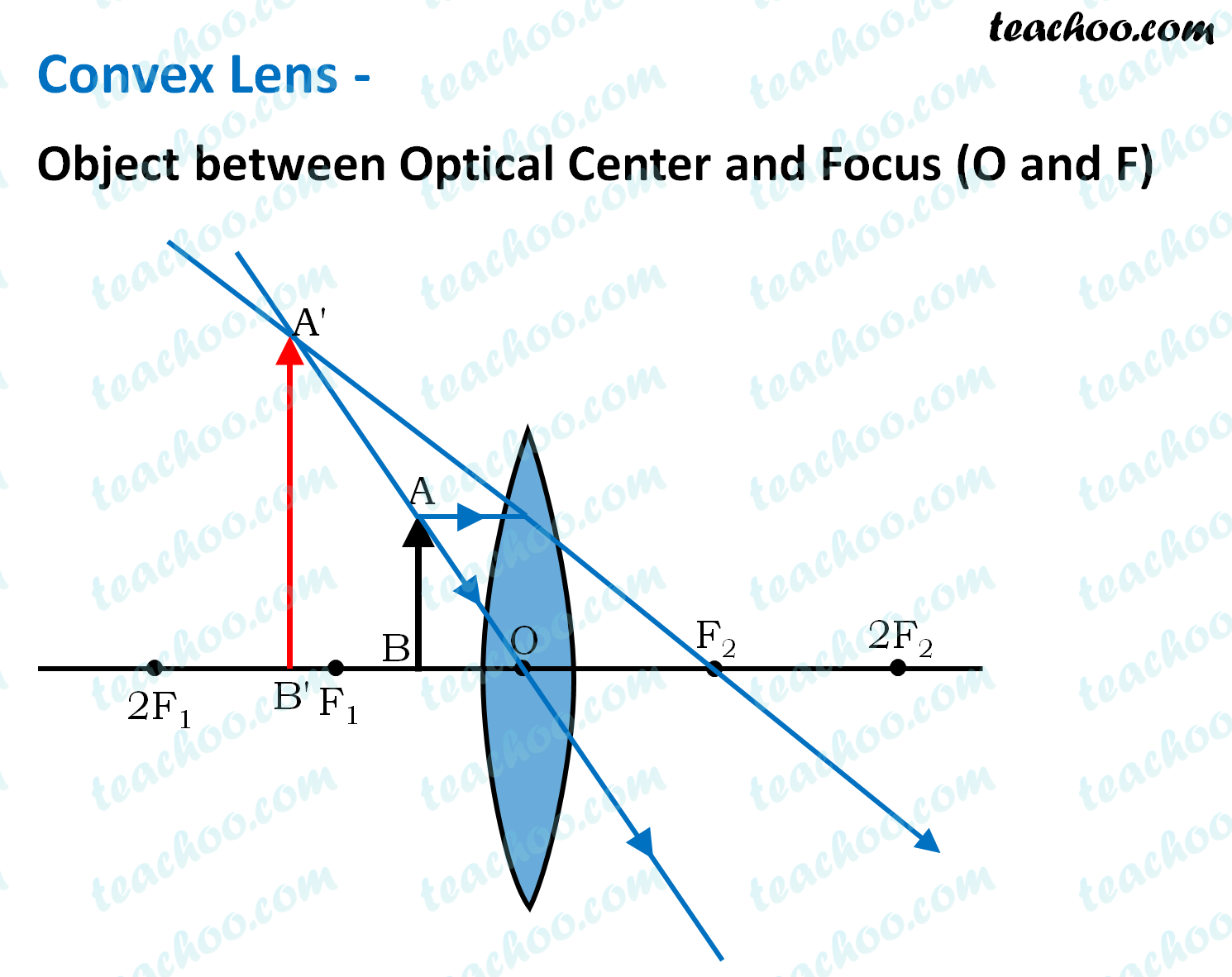Assertion Reasoning Questions (MCQ)

Class 10
Chapter 10 Class 10 - Light - Reflection and Refraction

## Reason (R): The convex lens can give diverging rays.

• It is not necessary that if the rays are diverging after emerging from the lens then the lens is concave.
• In Convex lens , if object is between O ptical Center and F ocus, the rays are diverging• If the rays crossing the focal point of the convex lens, become diverging.
• Therefore, lenses can be concave or convex.

Thus,

• Assertion is false
• Reasoning is true

So, the correct answer is (d)

Learn in your speed, with individual attention - Teachoo Maths 1-on-1 Class